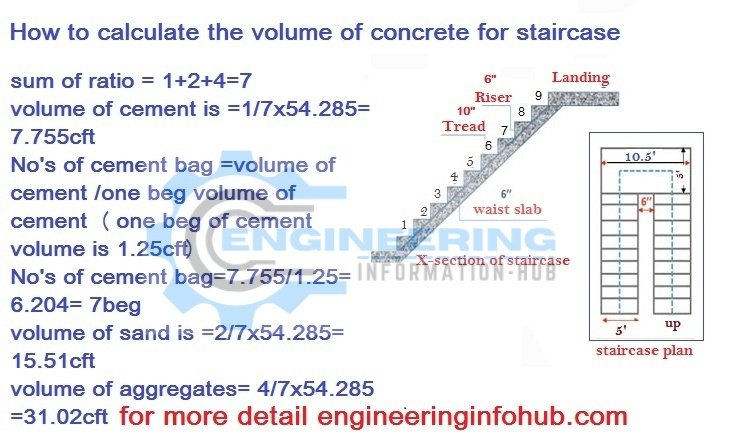# Estimate the Concrete Volume for the Staircase

Today I have the topic of the staircase | parts of staircase | what is The Staircase | Estimate the Concrete Volume for the Staircase. Calculate the Concrete Volume for the Staircase | Concrete For The Staircase

# What is The Staircase?

The staircase is the one part of the building that is used to help people to access any floor. now I find the concrete and also find the cement, sand, and aggregate. some different parts of a staircase.

step

Riser

flight

Landing

### Estimate the Concrete Volume for the Staircase

#### Now we start the calculation.Example :

The height up to the floor  = 10 ft

the height will 1 flight =  height of ist floor/ 2

height will 1 flight =  10/2    =  5ft

Riser                            =  6″ =  0.5′(ft)

Numbers  of riser                  =  height of one flight/height of the riser

Numbers  of riser                  = 5/0.5′  = 10

Number of tread                 =  no’s of riser -1

Number of tread                 = 10-1  =  9

thickness of diagonal slab   =  6″ = 0.5′(ft)

length of step               =  5′ (ft)

NOW

### The volume of concrete for step

the volume of one step =  riser×tread×length of step/2

volume 01 step = 0.5×0.833×5/2

volume 01 step = 1.041cft

we have 9 steps up to 01 floor and a volume of 1 step = 9×1=9cft

#### Now we calculate the volume of waste of the slab

ist find the length of the waist slab then find the volume of the diagonal slab.

we use the Pythagoras theorem

I know

the horizontal length of diagonal slab =tread×No’s of step

horizontal length of diagonal  slab =0.833×9 = 7.497cft

landing height from top of floor= no’s of riser  ×height of the riser

landings height from top of floor= 9 x 0.5′

landing height from top of floor= 4.5

NOW

length of waist slab =√horizontal distance of waist slab)² x √height of the landing from top to bottom )²

length of diagonal slab = √(7.497)² x √(4.5)²

length of diagonal slab = 8.744

The volume of concrete of diagonal slab= L × w × thickness of waist slab

the volume of concrete of diagonal slab= 8.744×5×0.5

the volume of concrete of diagonal slab=21.86cft

#### Now find the volume of concrete for landing of the staircase

length of landing =10.5′

width of landing  = 5′

Thickness of landing = 0.5′

The concrete volume of landing= 10.5×5×0.5

Concrete volume of landing=26.25cft

The total volume of concrete for the stair case=volume of ist flight+ volume of landing

### now total volume of the concrete for staircase is =9+26.25= 35.25cft

this volume of the concrete is wet for change wet to dry= multiplied by 1.54

the dry volume of the concrete of the staircase is = 35.25×1.54=54.285cft

##### Now we calculate the quantity of cement sand and aggregates

use ratio 1:2:4

sum of ratio = 1+2+4=7

volume of cement is =1/7×54.285= 7.755cft

No’s of cement bag =volume of cement /volume of one bag( volume of bag is 1.25cft)

No’s of cement bag=7.755/1.25= 6.204= 7beg

volume of sand is =2/7×54.285=15.51cft

volume of aggregates= 4/7×54.285=31.02cft

## THANKS.

### Raja Numan

Hi, My name is Engr. Raja Numan author of Engineering Information Hub and I am a Civil Engineer by Profession and I've specialized in the field of Quantity Surveying, Land Surveying as QC Engineer in national and multinational companies of Pakistan & Saudi Arabia.

### Related Articles

1.Ahmed says:
2.Kaleb Belihu Alemu says: